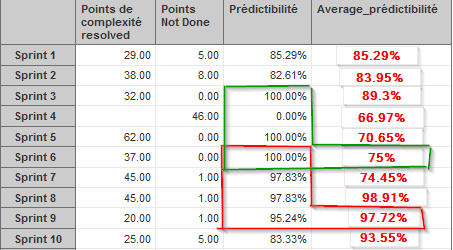# Determine for Each Sprint the Average Predictability for the last 4 Sprint

Hello,
I have an Agile report using Sprint dimension and i display on it :

• Points Done
• Points Not done
• Predictability ( Points Not Done / Point Done + not Done )

What i want to do is calculate for each Sprint the average predictability of this sprint and the 3 previous ones.

Result will be something like this :If someone can help me, i have read a lot of post that are quite similar but not exactly and i’m new using eazyBI.

Regards

Hi @Yannick

Welcome to the community!

You may want to reuse “Running velocity for 5 closed sprints” measure:

• instead of last 5 closed sprints, use last 3
• filter sprints by the existence of Story points at closing, not by committed points
• instead of “Sprint Story points completed” measure, use “Predictibilite” measure for the average calculation

The calculation formula would be the following (use correct measure “Predictibilite” with all specific characters):

``````CASE
WHEN
[Sprint].CurrentMember is [Sprint].DefaultMember
OR
-- for closed sprints only
[Sprint].CurrentMember.getBoolean('Closed') AND
NOT IsEmpty([Sprint].CurrentMember.get('Complete date')) AND
[Measures].[Sprint Story Points at closing] > 0
THEN
AVG(
Tail(
-- filter last 5 closed sprints starting from current sprint
Filter(
Cache(ChildrenSet([Sprint].[All closed sprints])),
Rank([Sprint].CurrentMember,
Cache(ChildrenSet([Sprint].[All closed sprints]))
)
),
-- only sprints with story points at closing are retrieved
[Measures].[Sprint Story Points at closing] > 0
), 3
),
[Measures].[Predictibilite]
)
END
``````

Notice!
In the report rows, use Sprint calculated member “All closed sprint” to align the set of sprint which is displayed in the report rows and used in the formula
You may want to add a filter in Pages by Project (it will also affect the set of the sprints in the formula)

Best,
Ilze, support@eazybi.com